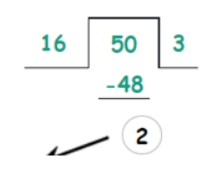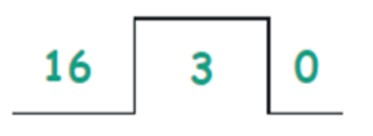# Binary to Hex Converter

Enter the binary number in the input box and hit calculate button to convert it into a hexadecimal number using binary to hex converter.

Error: UnKnown number
Swap

Give Us Feedback

Binary to hex converter is used to convert the binary number into a base 16 number system. This tool converts any binary number into hexadecimal numbers in a couple of seconds.

## What is a binary number?

A binary number is a base 2 number system in which only 0 & 1 integers are involved. For example, 10011 is a binary number of 19. All the numbers systems can be written in a binary number system.

## What is a hexadecimal number system?

A base 16 number system is known as a hexadecimal number system. There are 16 integers involved in this system of numbers. The numerical numbers from 0 to 15 are used in the base 16 number system.

It is abbreviated by the upper-case alphabets for the double-digit numbers. Such as A-F alphabets are used for denoting 10-15 numerals.

## How to convert binary to hex?

Following is a solved example of binary to hex conversion.

Example

Convert 110010 into a hexadecimal number system.

Solution

Step 1: First of all, convert the binary number to decimal.

(110010)2 = (1x25) + (1x24) + (0x23) + (0x22) + (1x21) + (0x20)
(110010)2 = (1x32) + (1x16) + (0x8) + (0x4) + (1x2) + (0x1)
(110010)2 = 32 + 16 + 0 + 0 + 2 + 0
(110010)2 = 50

Step 2: Convert decimal to the hexadecimal number system.

Divide the 50 by 16 to convert it from decimal to hex.

50/16The remainder of the division process is the rightmost digit of the hexadecimal number.

Now write the quotient in the numerator and divide it by 16 and repeat the process until it gives zero.The quotient will be zero as 3 is less than 16.

So, the hexadecimal number of 50 is 32.

### Math Tools